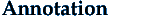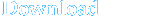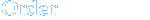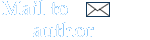The book System Modeling and Identification treats the methods of the building of the mathematical models of dynamic systems using the mathematical-physical modeling and the experimental identification. The application of the described methods is illustrated on the examples solved using the program MATLAB - Simulink. The book is written in Czech. The book is based on the author's experience from the teaching at the VSB - Technical University Ostrava, Faculty of Mechanical Engineering, Department of Control Systems and Instrumentation and his experience from the modeling and simulation of the industrial problems, especially from the modeling of the electrohydraulic drives and their technological applications.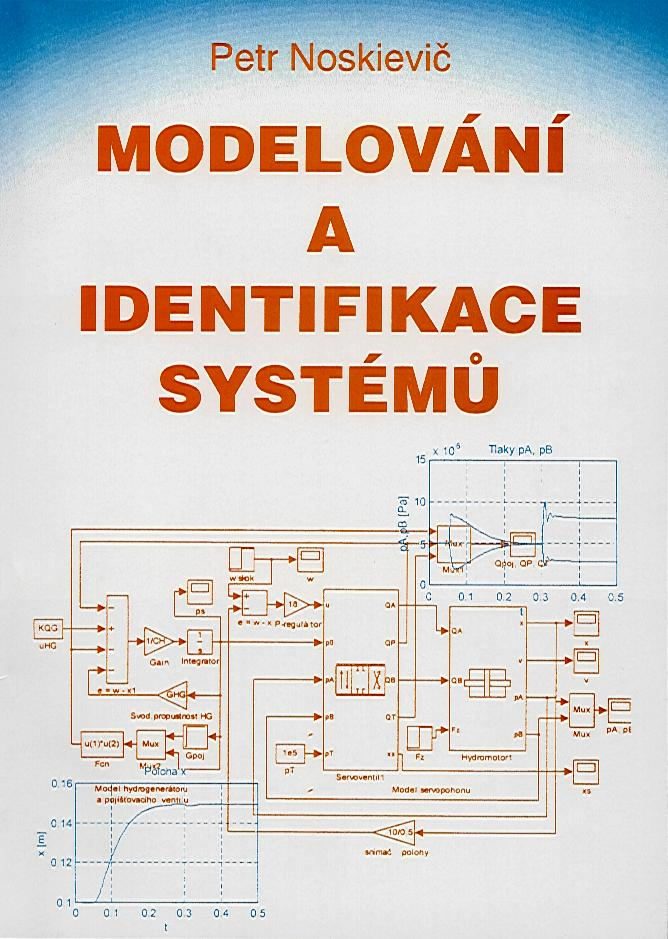The introductory chapter deals with the basic terminology of the systemtheory, mathematical and physical modeling. The principles and application of the modelingand simulation are illustrated in the case study. The mathematical-physical modeling of different systems is the topic ofthe following five chapters. The basic rules of the physical modeling of the real systemsof different kind - mechanical systems, electrical systems, hydraulic systems, pneumaticsystems and thermal systems - are given in the separate chapters. The derived mathematicalmodels are in the form of the differential equations, transfer functions and blockdiagrams. The topic modeling is summarized in the seventh chapter that deals with thephysical analogy. The methods of the experimental identification are the topic of thesecond part of the book. The used mathematical models and input signals are shown. Thecharacteristics of the basic linear dynamic systems are described. The methods used thedeterministic input signals are explained - how to approximate the step and impulseresponse of the system, how to build the step response from the impulse or ramp response.The methods for parametrization of the impuls and step response are described in the nextchapter. The statistic methods of the system identification are the topic of thenext three chapters. The basic statistic terms, the correlation methods and random inputsignals are explained. The system identification using the discrete model parametrization isdescribed in the next chapter. The construction of the process models AR, ARMA and dynamicsystems models ARX, ARMAX, Output Error and Box-Jenkins is explained. The parametrizationusing the least squares method and instrumental variable methods and their recursive formare derived. The identification of the systems operating in the closed loop is thetopic of the last chapter. The direct and indirect methods with and without test signalare shown. The printed scripts of the MATLAB commands and simulation models of theprogram Simulink could help with the study of the described methods. They could be alsovery helpful to the readers tasks solution. The book is intended for the students and engineers that are interestedin the modeling and identification of the dynamic systems. It gives the basic knowledgefrom the system modeling and identification. The explaining of the differentidentification methods could be very helpful to the application of the methods implementedin the Identification Toolbox and Signal Processing Toolbox of the MATLAB - Simulink.